## Finding Percentage Increase and Decrease

Practice Unlimited Questions

#### Word Problem 1: Justin bought a T-shirt at 12% off and received an additional membership discount of \$7. If the T-shirt cost him \$15, what was the original price of the T-shirt?\$15  +  \$7  =  \$22

The cost of the T-shirt before the membership discount was \$22.

100%  −  12%  =  88%

88%  →  \$22

1%  →
 \$22 88

100%  →
 \$22 88
×  100

=   \$25

The original price of the T-shirt was \$25.

#### Word Problem 2: 40% of the 7920 visitors to an amusement park were children. 25% of the children and 1 3 of the adults were repeat visitors. How many percent of the visitors were visiting the amusement park for the first time?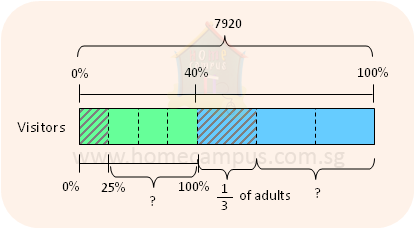40% of 7920  =
 40 100
×  7920

=   3168

There were 3168 children visiting the amusement park.

7920  −  3168  =  4752

There were 4752 adults visiting the amusement park.
25% of 3168  =
 25 100
×  3168

=   792

792 children were repeat visitors.
 1 3
of 4752  =
 1 3
×  4752

=   1584

792  +  1584  =  2376

There were 2376 repeat visitors.
7920  −  2376  =  5544

There were 5544 first-time visitors.
 5544 7920
×  100%  =  70%

70% of the visitors were visiting the amusement park for the first time.

#### Word Problem 3: Sarah had 55 cards. She gave 20% of them to her sister, 25% of the remaining to her brother and the rest to her best friend. How many percent of the cards did she give to her best friend?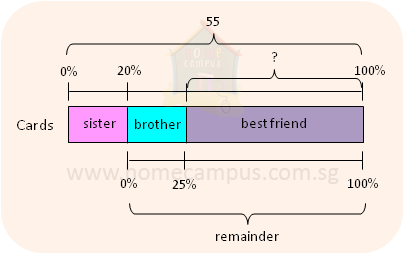Number of cards she gave to her sister  =  20% of 55

=
 20 100
×  55

=   11
Number of cards left for her brother and best friend  =  55  −  11

=   44
Number of cards she gave to her brother  =  25% of 44

=
 25 100
×  44

=   11
Number of cards she gave to her best friend  =  44  −  11

=   33
Percentage of cards she gave to her best friend  =
 33 55
×  100%

=   60%

She gave 60% of the total cards to her best friend.

#### Word Problem 4: 80% of the children in a class have pets. 45% of them have dogs, 40% have cats and the rest have birds. If 3 children in the class have birds, how many children are there in the class?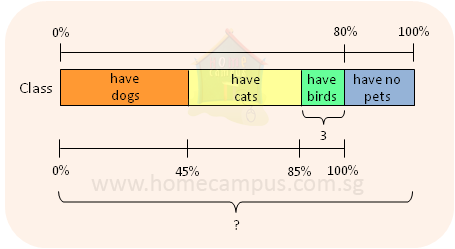100%  −  45%  −  40%  =  15%

15% of the children who have pets have birds.
15%  →  3

1%  →
 3 15

100%  →
 3 15
×  100

=   20

There are 20 children in the class who have pets.
80%  →  20

1%  →
 20 80

100%  →
 20 80
×  100

=   25

There are 25 children in the class.

#### Word Problem 5: A movie rental shop has 1375 movies for children. 40% of the movies are rated G while the rest are rated PG. The shop adds a few more G-rated movies to its collection increasing the number of G-rated movies to 45%. How many G-rated movies are added?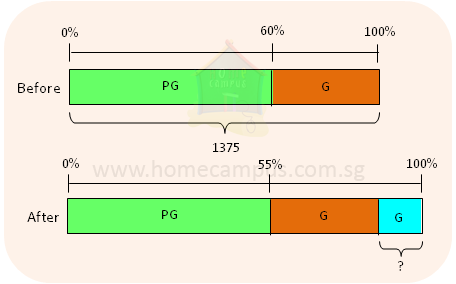100%  −  40%  =  60%

Before, the percentage of movies that are PG-rated is 60%.
Number of PG-rated movies  =  60% of 1375

=   60%  ×  1375

 60 100
×  1375

=   825

The number of PG-rated movies in the shop is 825.
100%  −  45%  =  55%

After more G-rated movies are added, the percentage of movies that are PG-rated reduces to 55%.
Now,

55%  →  825

1%  →
 825 55
=  15

100%  →  15 × 100  =  1500

There are now a total of 1500 movies.
Number of additional G-rated movies  =  1500  −  1375

=   125

There are 125 G-rated movies added.

#### Word Problem 6: Alice organized a yard sale of her old books on Saturday and Sunday, and expected to sell an equal number of books on each day. However, due to heavy rains on Saturday she could sell only 20% of her books that day. She managed to sell the rest of them on Sunday. Had she sold 36 more books on Saturday, she would have met her expectation. Find the number of books she sold altogether.100%  ÷  2  =  50%

50%  −  20%  =  30%

She had to sell 30% more books on Saturday to meet her expectation.
30%  →  36

1%  →
 36 30

100%  →
 36 30
× 100%

=   120

She sold 120 books altogether.

#### Word Problem 7: Grandma let Jon and Ron share her collection of seashells between them. Jon being the older of the two demanded a bigger share and took 160 seashells. Soon he realized he was being unfair. So, he gave 32 of his seashells to Ron which increased the number of seashells that Ron got by 25%. How many seashells were there in grandma's collection?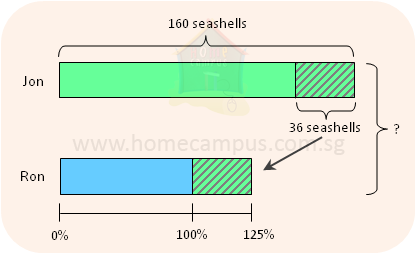25% of Ron's seashells  →  32

1% of Ron's seashells  →
 32 25

100% of Ron's seashells  →
 32 25
× 100%

=   128

Total number of seashells  =  160  +  128

=   288

There were 288 seashells in grandma's collection.

#### Word Problem 8: Erica has a sum of money that she is using to buy blouses at a clothing store. If she buys 2 similar blouses she will be short of 20% of the cost. If she buys 1 blouse she will have \$45 left. Find the cost of each blouse.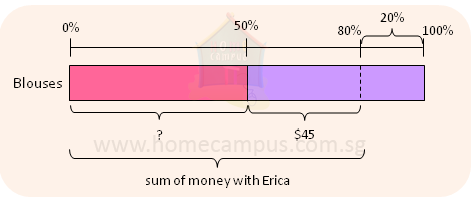30% of the cost of 2 blouses  →  \$45

1% of the cost of 2 blouses  →
 \$45 30

100% of the cost of 2 blouses  →
 \$45 30
× 100%

=   \$150

Cost of 1 blouse  =  \$150  ÷  2  =  \$75

The cost of each blouse is \$75.